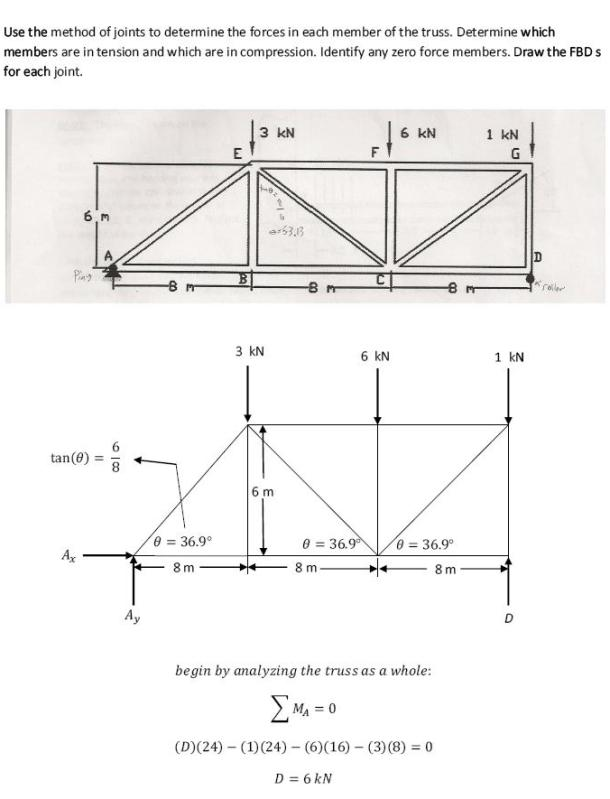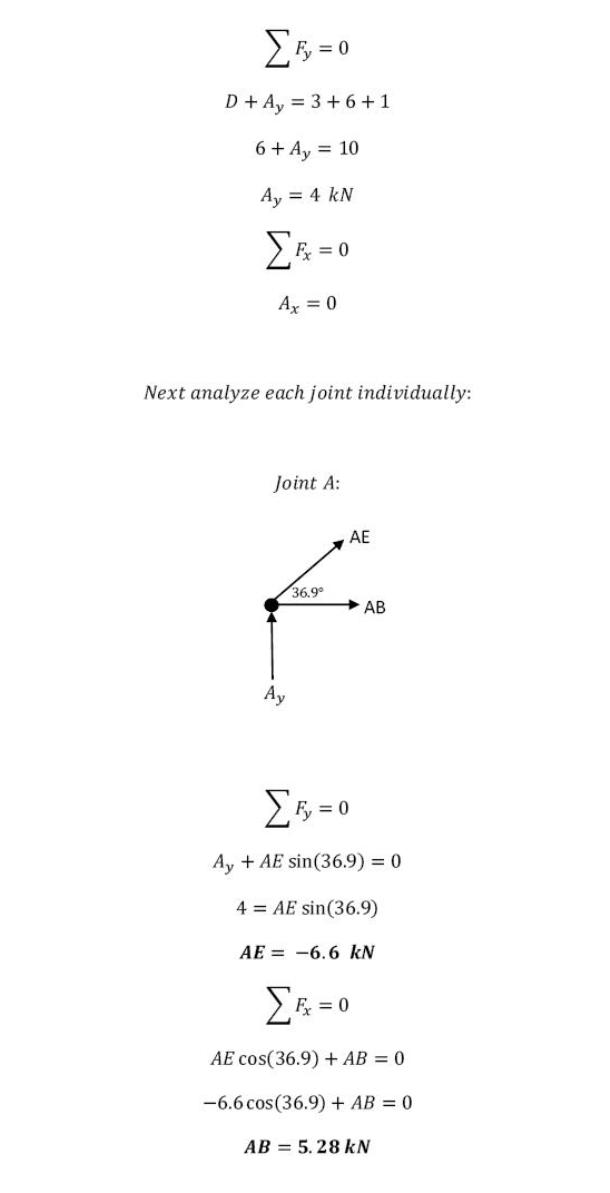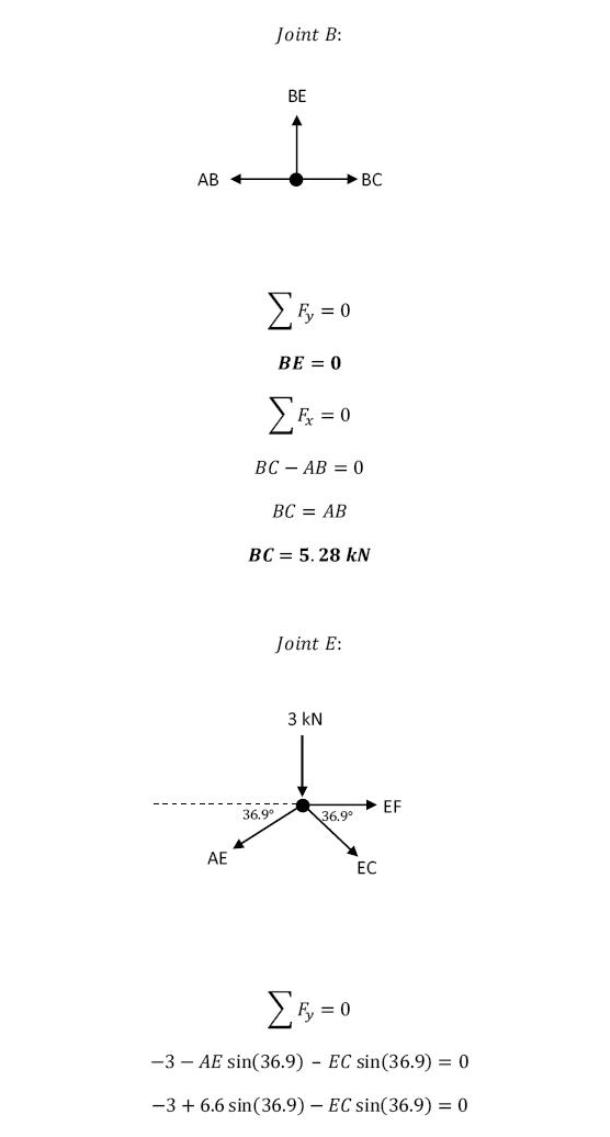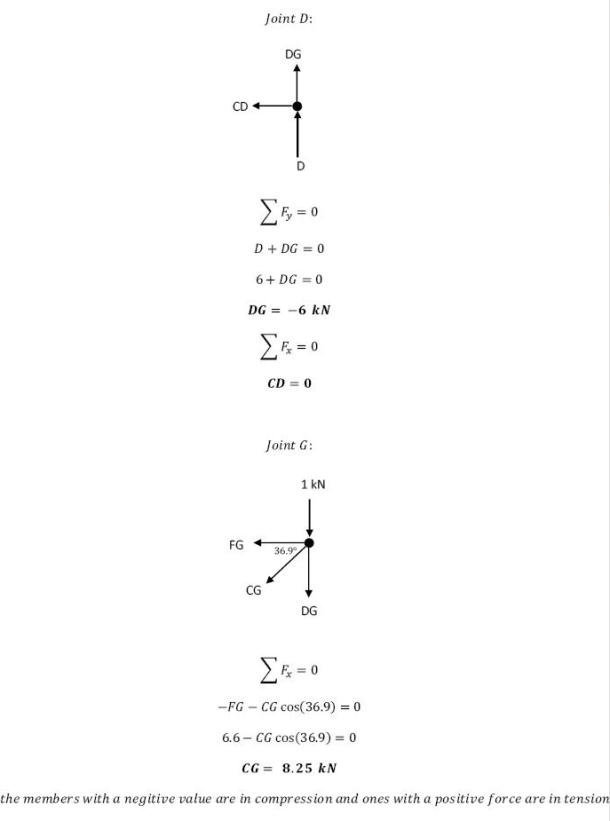statics math problems engineering equations statics basic mechanics school homework engineering math statics formulas statics problems statics problem solutions to statics problems full solution statics engineering statics problem solution statics math problems engineering equations statics basic mechanics school homework engineering math statics formulas statics problems statics problem solutions to statics problems full solution statics engineering statics problem solution
statics math problems engineering equations statics basic mechanics school homework engineering math statics formulas statics problems statics problem solutions to statics problems full solution statics engineering statics problem solution statics math problems engineering equations statics basic mechanics school homework engineering math statics formulas statics problems statics problem solutions to statics problems full solution statics engineering statics problem solution
Use the method of joints to determine the forces in each member of the truss. Determine which members are in tension and which are in compression. Identify any zero force members. Draw the FBD s for each joint.Use the method of joints to determine the forces in each member of the truss. Determine which members are in tension and which are in compression. Identify any zero force members. Draw the FBD s for each joint.